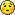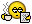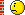ANNOUNCEMENT : ALL OF ROYAL MAIL'S EMPLOYMENT POLICIES (AGREEMENTS) AT A GLANCE (UPDATED 2017)... HERE

22 Jul 2011, 18:52

what is pareto?

i have heard the words "pegasus" and "georoute" but not pareto.

somebody been out shopping?

22 Jul 2011, 19:16

At a Pareto efficient allocation (on the Pareto frontier), the marginal rate of substitution is the same for all consumers. A formal statement can be derived by considering a system with m consumers and n goods, and a utility function of each consumer as zi = fi(xi) where is the vector of goods, both for all i. The supply constraint is written for . To optimize this problem, the Lagrangian is used:

where λ and Γ are multipliers.

Taking the partial derivative of the Lagrangian with respect to one good, i, and then taking the partial derivative of the Lagrangian with respect to another good, j, gives the following system of equations:

where ƒx is the marginal utility on ƒ' of x (the partial derivative of ƒ with respect to x).

22 Jul 2011, 19:19

wayoutman wrote:what is pareto?

Bet you wish you'd never asked now.
Ask your lino about it tomorrow. I'm sure he will be able to give you a simple explanation.

22 Jul 2011, 19:20

Also called the 80 / 20 law. 80% of worlds wealth is held by 20% of population. In RM 80% of the work is done by 20% of the workforce......possibly.22 Jul 2011, 19:25

80% of complaints probably do come from 20% of customers.

22 Jul 2011, 19:28

80% of the office moan about the other 20%

22 Jul 2011, 19:38

I've just read 20% of Postman's posts and 80% were rubbish.

22 Jul 2011, 20:42

80 % of bonuses go to less than 20 % of workforce

I'll call that the Parasite principle

22 Jul 2011, 21:11

80% of Avatars are ok,19.99 are okable 0.1 get...

23 Jul 2011, 13:16

jessicarabbit wrote:At a Pareto efficient allocation (on the Pareto frontier), the marginal rate of substitution is the same for all consumers. A formal statement can be derived by considering a system with m consumers and n goods, and a utility function of each consumer as zi = fi(xi) where is the vector of goods, both for all i. The supply constraint is written for . To optimize this problem, the Lagrangian is used:

where λ and Γ are multipliers.

Taking the partial derivative of the Lagrangian with respect to one good, i, and then taking the partial derivative of the Lagrangian with respect to another good, j, gives the following system of equations:

where ƒx is the marginal utility on ƒ' of x (the partial derivative of ƒ with respect to x).

aye. thats what i thought.

23 Jul 2011, 13:25

jessicarabbit wrote:At a Pareto efficient allocation (on the Pareto frontier), the marginal rate of substitution is the same for all consumers. A formal statement can be derived by considering a system with m consumers and n goods, and a utility function of each consumer as zi = fi(xi) where is the vector of goods, both for all i. The supply constraint is written for . To optimize this problem, the Lagrangian is used:

where λ and Γ are multipliers.

Taking the partial derivative of the Lagrangian with respect to one good, i, and then taking the partial derivative of the Lagrangian with respect to another good, j, gives the following system of equations:

where ƒx is the marginal utility on ƒ' of x (the partial derivative of ƒ with respect to x).

........... is this available in Welsh ?23 Jul 2011, 15:22

Pinky 1 wrote:
jessicarabbit wrote:At a Pareto efficient allocation (on the Pareto frontier), the marginal rate of substitution is the same for all consumers. A formal statement can be derived by considering a system with m consumers and n goods, and a utility function of each consumer as zi = fi(xi) where is the vector of goods, both for all i. The supply constraint is written for . To optimize this problem, the Lagrangian is used:

where λ and Γ are multipliers.

Taking the partial derivative of the Lagrangian with respect to one good, i, and then taking the partial derivative of the Lagrangian with respect to another good, j, gives the following system of equations:

where ƒx is the marginal utility on ƒ' of x (the partial derivative of ƒ with respect to x).

........... is this available in Welsh ?Be nice if it was available in English23 Jul 2011, 15:23

Bloody Hell Jessica expect a call from Rupert Murdoch, he needs someone to confuse them MPs.

24 Jul 2011, 01:27

Steviea34 wrote:
fishtank wrote:That's not individual performance stevie.....it's individual duty resourcing.
It's purely a "should take" measurement not a target that members have to meet.
It was never designed to measure performance.

Sorry fishtank, but what is the difference between
" should take time" and a performance measure.

It's the nueanced distinction we're reduced to making since we agreed to use IWT without getting movement from RM on their unreasonable performance standards first.clashcityrocker wrote:One is a conduct issue, the other isn't.

Thought it was a retraining issue?

24 Jul 2011, 06:21

Pinky 1 wrote:
jessicarabbit wrote:At a Pareto efficient allocation (on the Pareto frontier), the marginal rate of substitution is the same for all consumers. A formal statement can be derived by considering a system with m consumers and n goods, and a utility function of each consumer as zi = fi(xi) where is the vector of goods, both for all i. The supply constraint is written for . To optimize this problem, the Lagrangian is used:

where λ and Γ are multipliers.

Taking the partial derivative of the Lagrangian with respect to one good, i, and then taking the partial derivative of the Lagrangian with respect to another good, j, gives the following system of equations:

where ƒx is the marginal utility on ƒ' of x (the partial derivative of ƒ with respect to x).

........... is this available in Welsh ?Here you pinky in Welsh :

Ar dyraniad Pareto effeithlon (ar y ffin Pareto), y gyfradd ymylol y cyfnewid yr un fath ar gyfer yr holl ddefnyddwyr. Gall datganiad ffurfiol yn deillio drwy ystyried system gyda defnyddwyr m a nwyddau n, ac un o swyddogaethau gwasanaethau o bob defnyddiwr fel Zi = fi (xi) lle yn y fector o nwyddau, ar gyfer pob i. Mae'r cyfyngiad cyflenwad ei ysgrifennu ar gyfer. Er mwyn gwneud y gorau y broblem hon, mae'r Lagrangian yn cael ei ddefnyddio:

lle λ a Γ yn lluosyddion.

Gan gymryd y deilliad rhannol y Lagrangian mewn perthynas ag un da, i, ac yna cymryd y deilliad rhannol y Lagrangian o ran arall da, j, yn rhoi'r canlynol i'r system o hafaliadau:

lle ƒx yw'r cyfleustod ymylol ar ƒ 'x (deilliad rhannol ƒ mewn perthynas ag x).Page 2 of 5   1, 2, 3, 4, 5